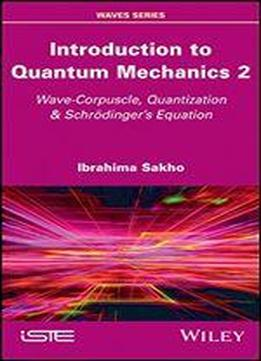# Introduction To Quantum Mechanics 2: Wave-corpuscle, Quantization & Schrdingers Equation by Ibrahima Sakho / 2020 / English / PDF

Quantum mechanics is the foundation of modern technology, due to its innumerable applications in physics, chemistry and even biology. This second volume studies Schrdingers equation and its applications in the study of wells, steps and potential barriers. It examines the properties of orthonormal bases in the space of square-summable wave functions and Dirac notations in the space of states. This book has a special focus on the notions of the linear operators, the Hermitian operators, observables, Hermitian conjugation, commutators and the representation of kets, bras and operators in the space of states. The eigenvalue equation, the characteristic equation and the evolution equation of the mean value of an observable are introduced. The book goes on to investigate the study of conservative systems through the time evolution operator and Ehrenfests theorem. Finally, this second volume is completed by the introduction of the notions of quantum wire, quantum wells of semiconductor materials and quantum dots in the appendices.

views: 160